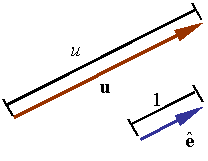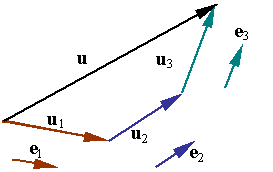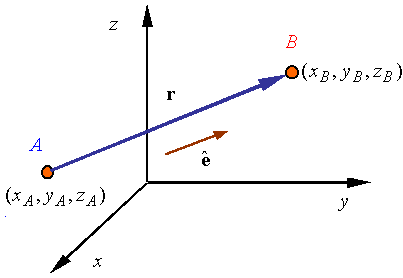×#### Thank you for registering.

One of our academic counsellors will contact you within 1 working day.

Click to Chat

1800-1023-196

+91-120-4616500

CART 0

• 0

MY CART (5)

Use Coupon: CART20 and get 20% off on all online Study Material

ITEM
DETAILS
MRP
DISCOUNT
FINAL PRICE
Total Price: Rs.

There are no items in this cart.
Continue Shopping```Vectors

Table of Content

Unit Vectors

Base Vectors and Vector Components

Negative of a Vector

Some Key Points

Some Basic Rules of VectorsVectors constitute an important topic in the Mathematics syllabus of JEE. It is important to master this topic to remain competitive in the IIT JEE. It often fetches some direct questions too. The topic of Vectors is quite simple and it also forms the basis of several other topics.

Various topics that have been covered in this chapter include:

Introduction

Fundamental Theorem of Vectors

Orthogonal System of Vectors

We now discuss some of these topics in brief as they have been covered in detail in the coming sections:

Length / Magnitude of a vector: The length or magnitude of the vector w = (a, b, c) is defined as

|w|= w= √a2+b2+c2

Unit Vectors

A unit vector is a vector of unit length. A unit vector is sometimes denoted by replacing the arrow on a vector with a "^" or just adding a "^" on a boldfaced character (i.e., ? or ?). Therefore,

| ?| = 1.

Any vector can be made into a unit vector by dividing it by its length.

? = u / |u|

Any vector can be fully represented by providing its magnitude and a unit vector along its direction. A vector can be written as u = u?You may refer the Sample Papers to get an idea about the types of questions asked.

Base Vectors and Vector Components

Base vectors represent those vectors which are selected as a base to represent all other vectors. For example the vector in the figure can be written as the sum of the three vectors u1, u2, and u3, each along the direction of one of the base vectors e1, e2, and e3, so that

u= u1+u2+u3It is clear from the figure that each of the vectors u1, u2 and u3 is parallel to one of the base vectors and can be written as a scalar multiple of that base. Let u1, u2, and u3 denote these scalar multipliers such that one hasu1= u1e1

u2=u2e2

u3=u3e3

The original vector u can now be written as

u= u1e1+u2e2+u3e3

Watch this Video for more reference

Negative of a Vector

A negative vector is a vector that has the opposite direction to the reference positive direction.A vector connecting two points:The vector connecting point A to point B is given by

r= (xB-xA) i+ (yB – yA) j + (zB – zA)k , here i, j and k denote the unit vectors along x, y and z axis respectively.

Some Key Points

The magnitude of a vector is a scalar and scalars are denoted by normal letters.

Vertical bars surrounding a boldface letter denote the magnitude of a vector.  Since the magnitude is a scalar, it can also be denoted by a normal letter;
|w| = w denotes the magnitude of a vector

The vectors are denoted by either drawing a arrow above the letters or by boldfaced letters.

Vectors can be multiplied by a scalar. The result is another vector.

Suppose c is a scalar and v = (a, b) is a vector, then the scalar multiplication is defined by cv= c (a,b)= (ca,cb). Hence each component of a vector is multiplied by the scalar.

If two vectors are of the same dimension then they can be added or subtracted from each other. The result is gain a vector. dd

Then the sum of these two vectors is defined by

v + u = (a + e, b + f, c + g).

We can also subtract two vectors of the same direction. The result is again a vector. As in the previous case subtracting vector u from v yields v - u = (a - e, b - f, c - g). the difference of these vectors is actually the vector

v - u = v + (-1)u.

Some Basic Rules of Vectors

If u, v and w are three vectors and c, d are scalars then the following hold true:

u + v = v + u (the commutative law of addition)

u + 0 = u

u + (-u) = 0 (existence of additive inverses)

c (du) = (cd)u

(c + d)u = cu +d u

c(u + v) = cu + cv

1u = u

u + (v + w) = (u + v) + w (the associative law of addition)

To read more, Buy study materials of Vectors comprising study notes, revision notes, video lectures, previous year solved questions etc. Also browse for more study materials on Mathematics here.
```### Course Features

• 731 Video Lectures
• Revision Notes
• Previous Year Papers
• Mind Map
• Study Planner
• NCERT Solutions
• Discussion Forum
• Test paper with Video Solution